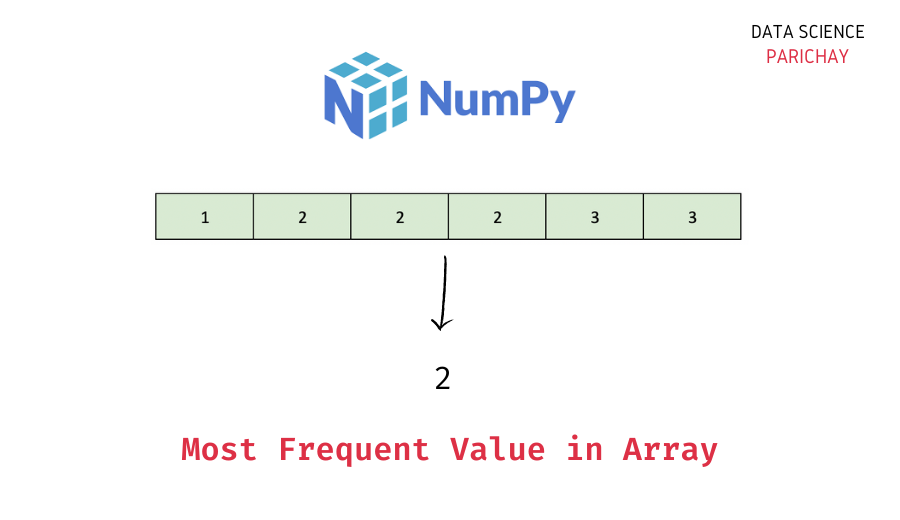# Get the Most Frequent Value in Numpy Array

The Numpy library in Python comes with a number of handy functions to work with arrays. In this tutorial, we will look at how to get the most frequent value in a Numpy array with the help of some examples.

## Steps to get the most frequent value in a Numpy array

To find the most frequent value in a Numpy array, find the count of each unique value in the array and then determine the most frequent value. Let’s take a look at a step-by-step example.

### Step 1 – Create a Numpy Array

First, we will create a Numpy array that we will be using throughout this tutorial.

```import numpy as np

# create numpy array
ar = np.array([1, 1, 2, 3, 4, 4, 4, 5])
# display the array
print(ar)```

Output:

`[1 1 2 3 4 4 4 5]`

Here, we used the `numpy.array()` function to creaate a Numpy array with some numbers. You can see that some values in the above array are repeated. The value 4 occurs three times, 1 occurs two times and the values 2, 3, and 5 occur once each in the above array.

### Step 2 – Find the unique values and their respective counts in the array

Use the `numpy.unique()` function to get all the unique values in the array. This function also gives you the count of each unique value in the array if you specify `return_counts = True` in the function call.

Let’s get the unique values and their respective counts in the above array.

```# get unique values and their respecitve frequency
vals, counts = np.unique(ar, return_counts=True)

# print the value and its frequecy
for val, count in zip(vals, counts):
print(f'{val} occurs {count} times')```

Output:

📚 Data Science Programs By Skill Level

Introductory

Intermediate ⭐⭐⭐

🔎 Find Data Science Programs 👨‍💻 111,889 already enrolled

Disclaimer: Data Science Parichay is reader supported. When you purchase a course through a link on this site, we may earn a small commission at no additional cost to you. Earned commissions help support this website and its team of writers.

```1 occurs 2 times
2 occurs 1 times
3 occurs 1 times
4 occurs 3 times
5 occurs 1 times```

We get an array of unique values and an array of counts. The value at the index `i` in the `counts` array represents the count of the value at the index `i` in the `vals` array.

### Step 3 – Get the most frequent value with the help of `argmax()`

We have the unique values and their counts. Now, to get the most frequent value, find the index of the maximum value in the `counts` array.

This index gives us the element with the highest frequency.

```# get the most frequent value
print(vals[counts.argmax()])```

Output:

`4`

We get 4 as the most frequent element which is the correct answer.

Here, we used the `numpy.ndarray.argmax()` function to get the index of the maximum value in the `counts` array and then used this index to find the most frequent value from the `vals` array.

## Summary – Find the most frequent element in Numpy array

In this tutorial, we looked at how to find the most frequent element in Numpy array. The following is short summary of the steps mentioned in this tutorial.

1. Create a Numpy array (skip this step if you already have an array to operate on).
2. Find the unique values and their respective counts in the array using the `numpy.unique()` function. Pass `return_counts = True`.
3. Use the Numpy `argmax()` function to find the index of the value with the highest count and use this index to find the most frequent value.

You might also be interested in –

•